# Section 7.6: Mathematics, Art, and Non-Euclidean Geometries

## 7.6 Outline

1. Golden rectangles
1. definition
2. divine proportion
3. tau
2. Mathematics and art
1. golden ratios in art
2. proportions of the human body
3. spiral constructed using a golden rectangle
3. Projective geometry
1. Duccio’s Last Supper
2. Hogarth’s Perspective Absurdities
3. false perspective
4. Masaccio’s The Holy Trinity
5. Durer’s Designer of the Lying Woman
4. Non-Euclidean geometry
1. Euclid’s fifth postulate
3. Lobachevskian postulate
4. hyperbolic geometry
5. pseudosphere
6. great circle
7. elliptic geometry
8. table showing comparisons of major two-dimensional geometries

## 7.6 Essential Ideas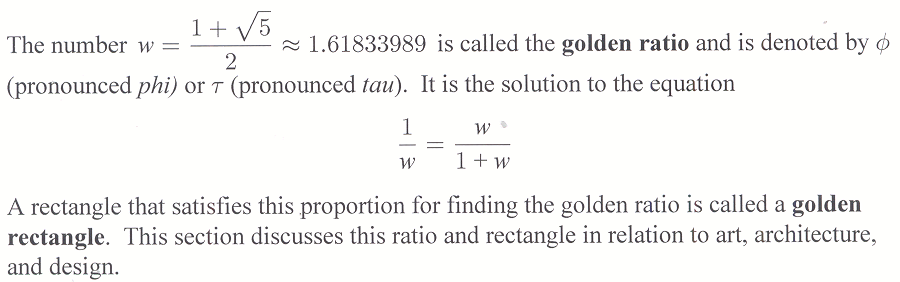A Saccheri quadrilateral has right angles as base angles and sides of equal length.

The summit angles may or may not be right angles.

The Lobachevskian Postulate: The summit angles of a Saccheri quadrilateral are acute.

This section discusses projective geometry and its relationship to three-dimensional representation in art. Next, non-Euclidean geometries are investigated with the idea in mind that Euclidean geometry is not the only possible geometry. The principle non-Euclidean geometries are hyperbolic and elliptic geometries.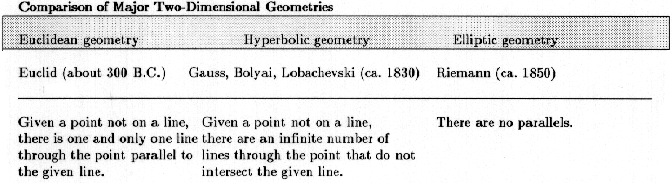A representative line in each geometry is shown in color for each models, and the shaded portion showing a Saccheri quadrilateral is shown directly below the respective models. Geometry is on a plane: Geometry is on a pseudosphere: Geometry is on a sphere: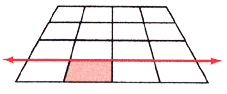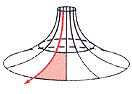The sum of the angles of a triangle is 180 degrees.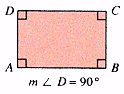Lines are infinitely long. The sum of the angles of a triangle is less than 180 degrees.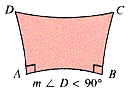Lines are infinitely long. The sum of the angles of a triangle is more than 180 degrees.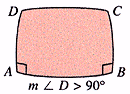Lines are finite in length.

The essential idea in classifying the correct geometry is the Lobachevskian postulate:
The summit angles of a Saccheri quadrilateral are acute.